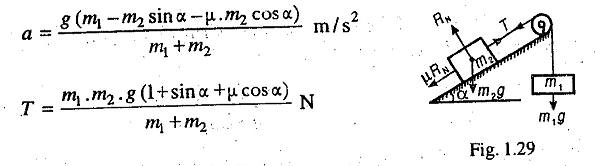# Motion of Two Bodies Connected by a String over a pulley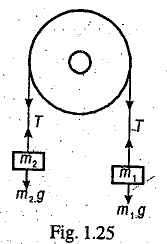Motion of Two Bodies Connected by a String over a pulley on different planes.

Consider a light inextensible string passing over a smooth pulley, as shown in Fig. 1.25, so that the tension (T) in both the strings is same.
Let mass m1 is greater than mass m2. Since the string is inextensible, the upward acceleration of mass m2 will be equal to the downward acceleration of mass m1. This acceleration is given by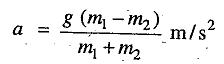and tension in the string,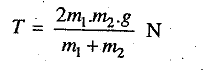Let us now consider the following cases of motion of two bodies connected by a string.

1. First of all, let us consider the motion of two bodies connected by an inextensible string, one of which is hanging freely and the other is lying on a smooth horizontal plane as shown in Fig. 1.26.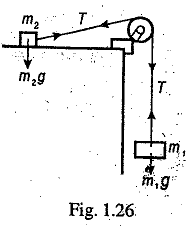Since the string is inextensible, the tension (T) in both the strings will be equal . The acceleration of the system is given by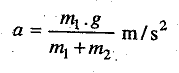and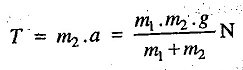2. If instead of smooth plane, it is a rough horizontal plane, as shown in Fig, 1.27, then frictional force equal to: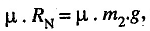will act in the opposite direction to the motion of mass m2, where u is the coefficient of friction. In such a case,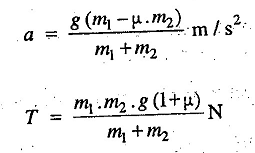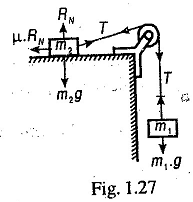3. When the plane is a smooth inclined plane, as shown in Fig. 1.28, then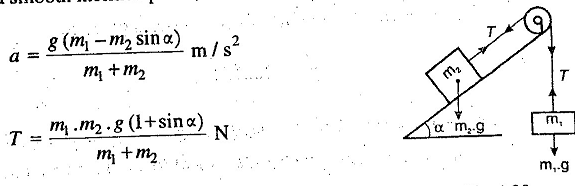4. When the plane is a rough inclined plane, as shown in Fig. 1.29, then